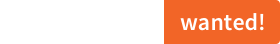# sciss / topology

A dynamic directed acyclic graph library. Mirror of https://git.iem.at/sciss/Topology

GitHub# Topology

## statement

Topology is a library for the Scala programming language that provides data structures and algorithms for graphs. It is (C)opyright 2010–2017 by Hanns Holger Rutz. All rights reserved. This project is released under the GNU Lesser General Public License v2.1+ and comes with absolutely no warranties. To contact the author, send an email to `contact at sciss.de`

## building

This project builds with sbt against Scala 2.12, 2.11.

To use this project as a library, use the following artifact:

``````libraryDependencies += "de.sciss" %% "topology" % v
``````

The current version `v` is `"1.1.0"`

## getting started

Instead of having a fixed vertex or edge type, most API uses an `EdgeView` type-class to obtain views from an opaque edge type to the vertices of th edge. There is a simply type `Edge` that can be used to generate plain edges from two vertices.

### Topology

The `Topology` type provides an online algorithm for maintaining a topologically sorted directed acyclic graph. The graph is embodied by the `Topology[V, E]` type where `V` is the opaque vertex type, and `E` is the directed edge type that implements the `Topology.Edge` trait, pointing to the source and target vertex. For example:

```    import de.sciss.topology._

case class Vertex(label: String)

// a new empty graph is constructed:
val t0 = Topology.empty[Vertex, Edge[Vertex]]```

The graph has methods `addVertex`, `removeVertex`, `addEdge` and `removeEdge` to evolve the topology. The structure is immutable, so calling any of these methods will return a new updated structure. The `addEdge` method is special in that it returns a `Try`. This is a `Failure` if the edge insertion could not be performed because it would produce a cyclic graph. The successful result is a tuple consisting of the new topology and an optional instruction to move affected vertices. This can be used, for example, by the call site to synchronize a linearized order to the vertices.

```    import scala.util.Success
import de.sciss.topology._

case class Vertex(label: String)

val t0 = Topology.empty[Vertex, Edge[Vertex]]

// m0 will contain the movement
val Success((t3, m0)) = t2.addEdge(Edge(Vertex("foo"), Vertex("bar")))
t3.addEdge(Edge(Vertex("bar"), Vertex("foo")))  // this is a failure
val t4 = t3.removeEdge(Edge(Vertex("foo"), Vertex("bar")))
// now it's possible. m1 will contain the movement
val Success((t5, m1)) = t4.addEdge(Edge(Vertex("bar"), Vertex("foo")))
val t6 = t5.removeVertex(Vertex("bar"))```

### Kruskal

Provides an algorithm to calculate a minimum-spanning-tree from a graph.

### Graph

Contains various utility methods for graphs.